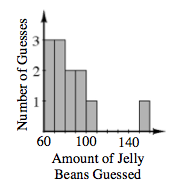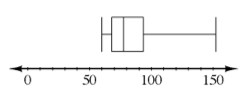### Home > ACC7 > Chapter 7 Unit 4 > Lesson CC2: 7.2.1 > Problem7-105

7-105.

Eduardo’s friends guessed how many jelly beans were in a jar at his birthday party. Here are their guesses: $75$, $80$, $95$, $92$, $100$, $72$, $71$, $60$, $65$, $88$, $60$, $152$.

1. Make a histogram to display the data.

Make the $x\text{-axis}$ ''Jelly Beans'' and the $y\text{-axis}$ ''Guesses''. How would you want to scale the $x\text{-}$ and $y\text{-axes}$?2. Make a box plot to display the data.

Find the start, first quartile, Median, third quartile, and the end.3. Which measure of center is most appropriate for this data? Why?

Considering the outlier, would the mean or the median be most appropriate?

4. Which data display most clearly shows the median of the data?

Which plot takes into account the median when it is being constructed?